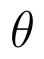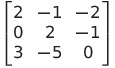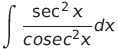Select Page

# Maths 12 Science CBSE Three Dimensional Geometry Answers for MCQ in English

Maths 12 Science CBSE Three Dimensional Geometry Answers for MCQ in English to enable students to get Answers in a narrative video format for the specific question.

Expert Teacher provides Maths 12 Science CBSE Three Dimensional Geometry Answers for MCQ through Video Answers in English language. This video solution will be useful for students to understand how to write an answer in exam in order to score more marks. This teacher uses a narrative style for a question from Three Dimensional Geometry not only to explain the proper method of answering question, but deriving right answer too.

Please find the question below and view the Answer in a narrative video format.

Question:

## Similar Questions from CBSE, 12th Science, Maths, Three Dimensional Geometry

Question 1 : Find the distance of the point (2, 12, 5) from the point of intersection of the linesand the plane(View Answer Video)

Question 2 : Find the co-ordinates of the point where the line through the points A(3, 4, 1) and B(5, 1, 6) crosses the XY-plane. Also find angle which this line makes with the XZ-plane. (View Answer Video)

Question 3 : Find the distance of the point (1, -2, 3) from the plane x-y+z=5 measured parallel to the line(View Answer Video)

Question 4 : If a line marks anglesandwith x, y and z-axis respectively, whereis acute, then find. (View Answer Video)

Question 5 : If a lines makes angleandwith the positive directions of x-axis and z-axis respectively, then find the angle that it makes with the y-axis. (View Answer Video)

### Determinant

Question 1 :  Evaluate the determinants:. (View Answer Video)

Question 3 : Let A be a non-singular square matrix of order 3 * 3. Then | adj A | is equal to: (View Answer Video)

Question 4 : If the area of a triangle is 35 sq units with vertices (2, -6), (5, 4) and (k, 4). Then k is: (View Answer Video)

### Integrals

Question 1 : Find:. (View Answer Video)

Question 2 : Evaluate :(View Answer Video)

Question 3 :(View Answer Video)

Question 4 : Find:. (View Answer Video)

Question 5 : Write the value of:(View Answer Video)

### Probability

Question 1 : A die is thrown 6 times. If 'getting an odd number' is a 'success', what is the probability of obtaining:
exactly 5 successes?
Question 2 : Ifandfindif E and F are independent events.  (View Answer Video)
Question 5 :  A die is rolled. If E= (1, 3, 5), F(2, 3) and G= {2, 3, 4, 5}, find(View Answer Video)